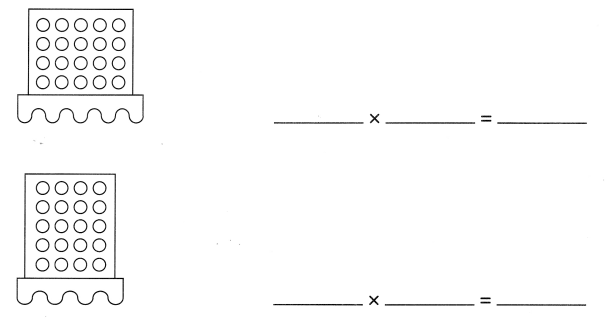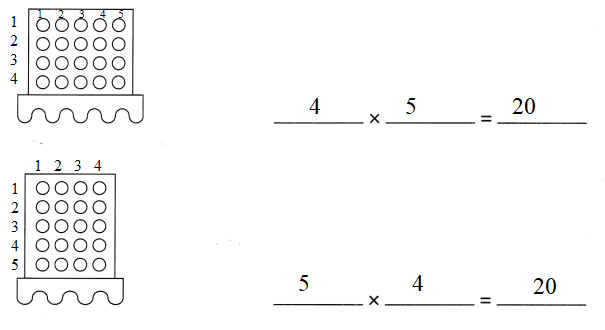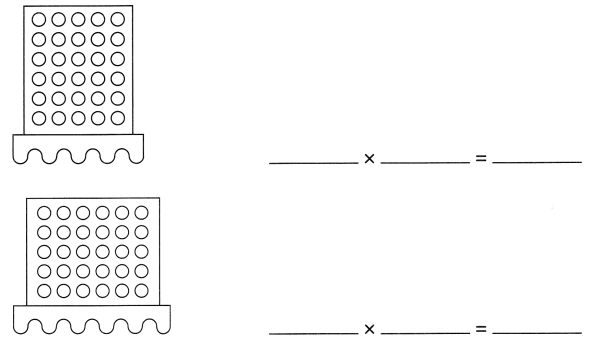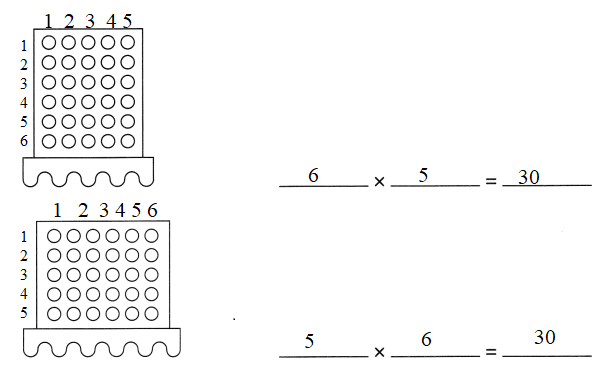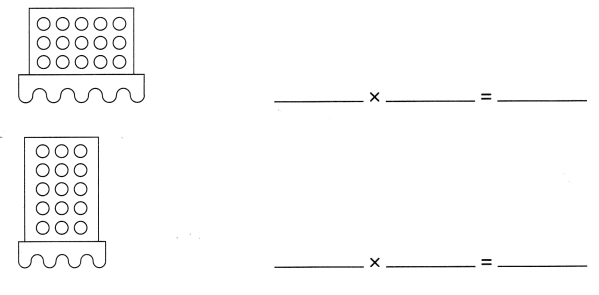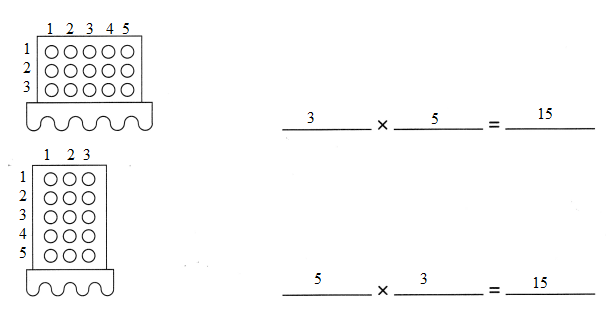# Math in Focus Grade 2 Chapter 6 Practice 4 Answer Key Multiplying 5: Using Dot Paper

Go through the Math in Focus Grade 2 Workbook Answer Key Chapter 6 Practice 4 Multiplying 5: Using Dot Paper to finish your assignments.

## Math in Focus Grade 2 Chapter 6 Practice 4 Answer Key Multiplying 5: Using Dot Paper

Use dot paper to solve.

Example
Kelly uses 5 beads to make one necklace. How many beads does she use to make 8 necklaces?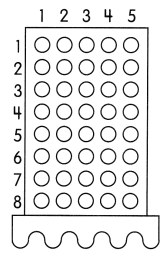8 × 5 = 40
She uses 40 beads to make 8 necklaces.

Question 1.
9 friends buy tickets to a movie. Each ticket costs $5. How much do the friends pay in all for the tickets? _____ ×$ ____ = $____ The pay$_____ in all for the tickets.
9 × $5 =$45
The pay $45 in all for the tickets. Explanation: Given: Number of friends are there to by tickets = 9 friends each tickets cost =$5
To know how much the friends have to pay in all for the tickets, we need to multiply 5 with 9 then we get
5 × 9 = 45
So, they have to pay \$45 in total for the tickets

Question 2.
Ellen packs some books into 10 boxes. She packs 5 books into each box. How many books does she pack in all?
_____ × _____ = _____
She packs ____ books into 10 boxes.
10 × 5 = 50
She packs 50 books into 10 boxes.
Explanation:
Given:
Total number of boxes Ellen packs the books into = 10
In each box she packs = 5 books
To know how many hooks in total she packs we need to multiply 10 with 5 so we get
10 × 5 = 50
In total she packs 50 books in 10 boxes.

Multiply.

Example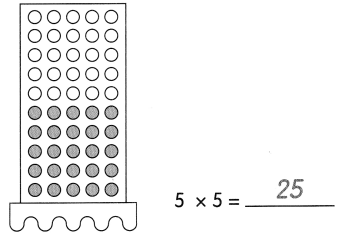Question 3.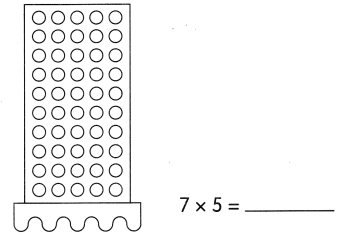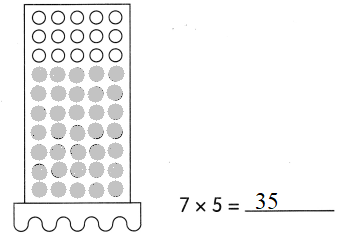Multiply.

Question 4.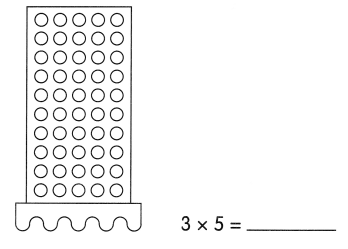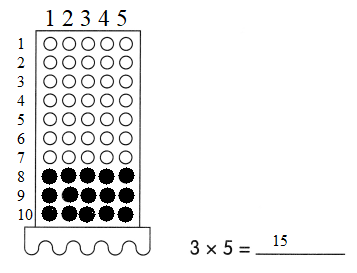Use dot paper to fill in the blanks.

Question 5.